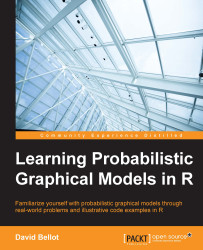•#### Learning Probabilistic Graphical Models in R#### Overview of this book

Probabilistic graphical models (PGM, also known as graphical models) are a marriage between probability theory and graph theory. Generally, PGMs use a graph-based representation. Two branches of graphical representations of distributions are commonly used, namely Bayesian networks and Markov networks. R has many packages to implement graphical models. We’ll start by showing you how to transform a classical statistical model into a modern PGM and then look at how to do exact inference in graphical models. Proceeding, we’ll introduce you to many modern R packages that will help you to perform inference on the models. We will then run a Bayesian linear regression and you’ll see the advantage of going probabilistic when you want to do prediction. Next, you’ll master using R packages and implementing its techniques. Finally, you’ll be presented with machine learning applications that have a direct impact in many fields. Here, we’ll cover clustering and the discovery of hidden information in big data, as well as two important methods, PCA and ICA, to reduce the size of big problems.
Learning Probabilistic Graphical Models in RCreditswww.PacktPub.comPrefaceFree Chapter
Probabilistic ReasoningExact InferenceLearning ParametersBayesian Modeling – Basic ModelsApproximate InferenceBayesian Modeling – Linear ModelsProbabilistic Mixture ModelsAppendixIndex## Latent Dirichlet Allocation

The last model we want to present in this book is called the Latent Dirichlet Allocation. It is a generative model that can be represented as a graphical model. It's based on the same idea as the mixture model, with one notable exception. In this model, we assume that the data points might be generated by a combination of clusters and not just one cluster at a time, as was the case before.

The LDA model is primarily used in text analysis and classification. Let's consider that a text document is composed of words making sentences and paragraphs. To simplify the problem, we can say that each sentence or paragraph is about one specific topic, such as science, animals, sports, and so on. Topics can also be more specific, such as cats or European soccer. Therefore, there are words that are more likely to come from specific topics. For example, the word cat is likely to come from the topic cats. The word stadium is likely to come from the topic European soccer. However...